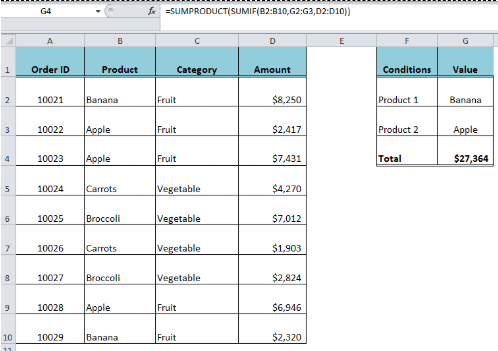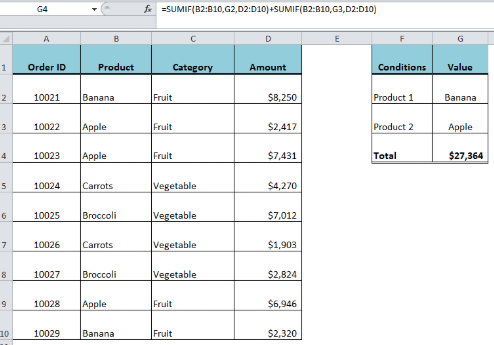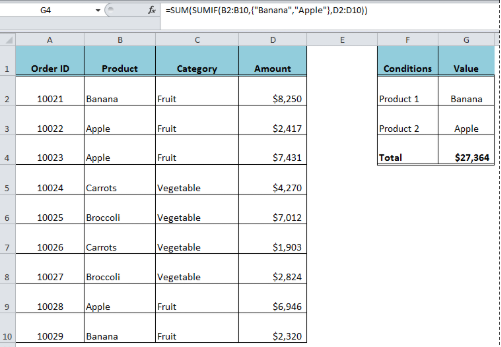Get instant live expert help with Excel or Google Sheets“My Excelchat expert helped me in less than 20 minutes, saving me what would have been 5 hours of work!”

#### Post your problem and you’ll get Expert help in seconds.

Your message must be at least 40 characters
Our professional Expert are available now. Your privacy is guaranteed.

# How to Use the SUMIF and OR Functions Together

The SUMIF function in Excel is used to sum values based on a single condition or criteria. However, if we want to sum values based on multiple criteria where at least one of the conditions are met, we use the SUMIF with OR logic. There are different methods to do this.Figure 1. Final result

We sum the results of multiple SUMIF functions in one cell where each SUMIF function handles one condition or criteria. In our dataset, we have a table of products’ orders and their values. We need to add up the orders’ values of two products “Banana” and “Apple”, whichever is available using SUMIF with OR criteria in the following formula;

`=SUMIF(B2:B10,"Banana",D2:D10)+SUMIF(B2:B10,"Apple",D2:D10)`

OR

`=SUMIF(B2:B10,G2,D2:D10)+SUMIF(B2:B10,G3,D2:D10)`Figure 2. Adding multiple SUMIF functions

## Using SUM and SUMIF with an Array Constant

Here, we supply more than one value of the criteria using an array constant in criteria argument of the SUMIF function. An Array constant is a list of criteria values, separated by commas, and this list of values is enclosed in curly brackets. As we have more than one value as criteria in the array constant, so the SUMIF function will return one result for each value of criteria in the array. Therefore, we finally wrap the SUMIF function in the SUM function to add up the results of each criterion based on the SUMIF with OR criteria.

`=SUM(SUMIF(B2:B10,{"Banana","Apple"},D2:D10))`

Note that the criteria values in the array constant are supplied directly and not as a cell reference. The text values are supplied in double quotation marks, and numeric values are supplied without double quotation marks.Figure 3. Using SUM and SUMIF function with an array constant

## Using SUMIF with SUMPRODUCT Function

If we want to list the criteria values in cell references rather than supplying them directly in the formula, we wrap the SUMIF function in the SUMPRODUCT function. The criteria values are listed in the range of cells and then we supply this range in the criteria argument of the SUMIF function. The SUMIF function returns the result for each criteria value as an array of values and the SUMPRODUCT returns the sum of those resulting values based on the SUMIF with OR criteria. In this example, the values of the products are listed in range G2:G3 as criteria argument in the SUMIF function in the following formula.

`=SUMPRODUCT(SUMIF(B2:B10,G2:G3,D2:D10))`Figure 4. Using SUMIF with SUMPRODUCT function

## Instant Connection to an Expert through our Excelchat Service:

Most of the time, the problem you will need to solve will be more complex than a simple application of a formula or function. If you want to save hours of research and frustration, try our live Excelchat service! Our Excel Experts are available 24/7 to answer any Excel question you may have. We guarantee a connection within 30 seconds and a customized solution within 20 minutes.

### Did this post not answer your question? Get a solution from connecting with the expert.Another blog reader asked this question today on Excelchat:
Solution examplesI am looking for a formula to SUM column L, if Column A matched the relevant date and column C matches the name
Solved by Z. Y. in 16 minsHi, I'm making myself a weekly budget spreadsheet. I've made a sheet with all my monthly outgoings, and I've worked out how to use SUMIF to combine the amounts for each day, but I want to include a reference and don't know how to combine text cells based on a specific date reference... does that make sense?
Solved by E. U. in 60 minsThe formula is: =SUMIF(BAARCH!A:R,summary!A6,BAARCH!P:P) which searches for the name inputted on the summary sheet on the BAARCH worksheet - but I want it to look for the name across a range of worksheets. The column is PP.
Solved by Z. C. in 50 minsI need a formula that will check if 2 or more cells containing names match then add the adjacent cells to matching name together.
Solved by F. D. in 59 minsI need shorthand to reference the column in the row im working on. For instance instead of writing =SUMIF(Table2[ITEM CODE],B8,Table2[QTY]) I want to write something like =SUMIF(Table2[ITEM CODE],Column(B),Table2[QTY])
Solved by C. C. in 20 mins## Subscribe to Excelchat.coAnother blog reader asked this question today on Excelchat: